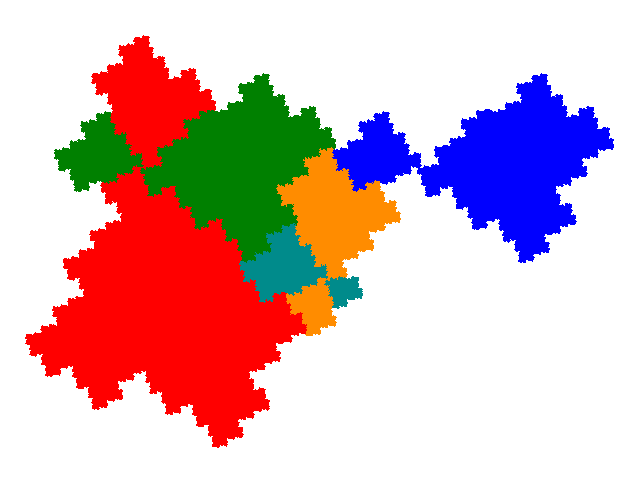# Some tiles associated with the 6th unit cubic Pisot number

## Order 5 and Order 7 Parametasymmetric Tiles (Overview)

Applying the partial postcomposition technique to the order 3 symmetric tile produces some symmetric and some asymmetric tiles. The grouped element technique can then be applied to the symmetric tiles generating many new tiles.For any order 5 tile there are 32 attractors, including the original one. The same set of attractors is generated regardless of which asymmetric tile is started with. 8 of the attractors generate 6 partial postcomposition derivatives of the symmetric and demisymmetric tiles, and one is disconnected, leaving 23 novel tiles. It turns out that all of these 23 tiles are fractional symmetric tiles, where 4 copies, made up of two copies each of two different sizes make up a symmetric tile. The cell transforms are `p→p` `p→-p` `p→ap - 1` `p→-(ap - 1)` The unit cells are shown on the pages for the various groups of tiles. While all of these are generated by application of the co-cell technique to the assymmetric order partial postcomposition derivatives of the symmetric tile, the 11 tiles (and 1 disconnected figure) making up the first and third groups above are also obtained by partial postallocomposition of the degenerate attractors of the symmetric tile.For any order 7 tile there are 128 attractors, including the original one. The same set of attractors is generated regardless of which asymmetric tile is started with. In this case only 3 of the attractors generate already known tiles, but a greater proportion are disconnected, resulting in a total of 82 novel tiles. It turns out that all of these 82 tiles are fractional symmetric tiles, where 6 copies, made up of two copies each of three different sizes make up a symmetric tile. The cell transforms are `p→p` `p→-p` `p→ap - 1` `p→-(ap - 1)` `p→ap3` `p→-ap3` The unit cells are shown on the pages for the various groups of tiles.

© 2015, 2016 Stewart R. Hinsley